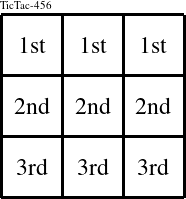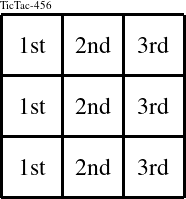Detailed instructions for working a group puzzle of type 'TicTac-456'

The 3 elements in each group of this type of puzzle are: 4, 5, and 6.

Every group in the puzzle must contain exactly this set of elements.

####In a TicTac-456 puzzle, each row is a group numbered as shown in the figure above.

####In a TicTac-456 puzzle, each column is a group numbered as shown in the figure above.